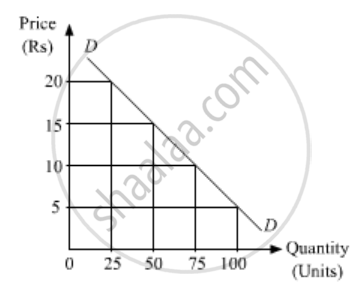# Explain the Law of Demand with Its Assumptions. - Economics

Explain the law of demand with its assumptions.

#### Solution 1

Assumptions of The Law of Demand:-

The Law of Demand is based on the following assumption.

1. Size and composition of the population remains constant:-

There should not be any change in the size and composition of the population. Because a change in population will bring about a change in demand even if the price remains the same.

2. The income of the consumer remains constant:-

The income of consumer should remain constant. If there is any change in income, demand tends to change even though the price is constant. For example, if income increases people will demand more quantity of a commodity even at a higher price.

3. Tastes and habits remain constant:-

Taste, habit, custom, tradition, and fashion, etc. should remain unchanged. Due to changes in taste and preference, people's demand for goods undergoes a change.

4. No change in expectations about future price changes:-

There should not be any change in the expectations about the prices of, goods in the future. If consumers expect that price will rise or fall in the future, they will change their present demand though price is.constant.

5. Prices of substitutes and, complementary goods remain constant:-

The prices of substitute and complementary goods should remain constant. For instance, if the price of tea rises, its demand will fall but demand for coffee will increase.

6. Government Policy remains constant:-

The taxation and fiscal policy of the government should not change. A change in income tax, for instance, may cause changes in consumer's disposable income and hence demand.

#### Solution 2

The law of demand is based on the following assumptions:

i. The size of the population remains the same.
ii. The income of the consumer remains unchanged.
iii. Prices of related goods remain unchanged.
iv. Consumer’s tastes and preferences remain unchanged.
v. The government’s policies remain unchanged.
vi. There is no change in expectations about the future.
The law of demand can be explained with the help of the following demand schedule
 Price of Commodity (X)(Rs) Quantity Demanded of X(Units) 5 100 10 75 15 50 20 25

The schedule shows that as the price of commodity X increases from Rs 10 to Rs 15, the quantity demanded X falls from 75 units to 50 units. Thus, there is a negative relationship between demand and price.Let us plot the above schedule on a graph. On the x-axis, we represent the quantity demanded; and on the y-axis, we represent the price of the good. Joining the different combinations of price and quantity demanded, we get a curve DD. This curve is the demand curve showing the inverse relationship between the price and the quantity demanded.

Is there an error in this question or solution?
2015-2016 (March)

Share